## Tuesday, June 28, 2016

### Fix for bug in `collapseTree`

Not long after submitting a new version of phytools (phytools 0.5-38) to CRAN, which has been accepted, I discovered a weird bug in the interactive function `collapseTree`. `collapseTree` is interactive, so it is kind of hard to demo; however, a video of it's use can be seen here.

The bug is due to the plotrix function `draw.circle` either no longer being vectorized or not being correctly vectorized. I essentially fixed this by substituting:

``````draw.circle(x,y,radius=r,border=col,col="white",nv=20)
``````

for:

``````nulo<-mapply(draw.circle,x=x,y=y,radius=r,MoreArgs=list(border=col,
col="white",nv=20)
``````

This fix is on GitHub and can be installed in the typical way.

## Friday, June 24, 2016

### New version of phytools (0.5-38) on CRAN

There is a new version of phytools (0.5-38) on CRAN in advance of the workshop I will teach this coming week in San Juan, Puerto Rico with Mike Alfaro, Ricardo Betancur, and Luke Harmon.

Here are some of the updates for this version:

1. A simple function (`matchLabels`) to match labels between trees.

2. A new function (`consensus.edges`) containing various methods to compute the consensus edge lengths from a set of trees.

3. Fixes to the functions `dotTree` and `fitPagel` (here). (Also here: 1, 2.)

4. An update to `evol.vcv` to fit a one matrix only model. (I also subsequently pushed an update to allow it work for only one trait too.)

5. New S3 method updates for object classes `"simmap"` and `"multiSimmap"` and new function `mapped.states` (here).

6. More important fixes to `dotTree` for trees containing few taxa.

7. Some internal (but important) ways in which the way phytools plots left-facing trees. (Also here.)

8. New function, `averageTree` for computing the 'average' phylogeny in a set, by some criteria (1, 2, 3, 4).

9. Separate user control of left- and right-facing tree font sizes in `plot.cophylo` (here).

10. Update to `cophylo` to permit links in `assoc` for taxa not found in the trees (here).

11. Simple `as.phylo` method for objects of class `"simmap"` and `"multiSimmap"` (here).

12. New options for setting the root state in `sim.history`.

13. A version of `bind.tree` for objects of class `"simmap"` (also here).

14. Important updates to `fitPagel` to permit different dependency relationships between traits and different models of evolution.

15. New options for user control in `dotTree` (here).

16. A new S3 plot method for objects of class `"fitPagel"` (1, 2).

17. S3 plotting method for discrete character models fit using `fitMk` or `fitDiscrete` in the geiger package (1, 2, 3).

18. Important updates to the `plot.phylo.to.map` method (here).

19. Update to function (`multiC`) for computing multiple phylogenetic VCV matrices depending on the state of a mapped trait (here).

20. Important bug fix for the function `contMap`.

21. Update to `roundPhylogram` to permit different line edges and types.

22. Finally, a new interactive version of `phylo.toBackbone` to permit a backbone tree with subtrees plotted as triangles to be created interactively, rather than via a static translation table (here).

These updates are already on CRAN - but it will probably take a couple of days for binaries to be built and percolate through the mirror repositories. In the meantime, as always, phytools can be installed from GitHub. Enjoy!

### Interactive version of `phylo.toBackbone`

I just created a new interactive version of the phytools function `phylo.toBackbone`, which creates a backbone phylogeny in which some clades are represented as triangles. This tree can then we plotted with an S3 `plot` method for the object class `"backbonePhylo"`. Most of the code changes can be seen here. The updates are kind of hacky - principally because the original version of this appears to have been coded by me in 2013.

In previous versions of this function (e.g., 1, 2) the user had to supply the backbone tree as input along with a translation table. This is still possible, or course; however the current version allows the user to collapse clades into triangles by clicking on the fully plotted tree. This should be a nice feature for some users.

Obviously, interactive mode is kind of difficult to demonstrate - consequently I have embedded a screenshot video of a demo at the bottom. The following is just an illustration of the product. Remember for publication to save to PDF (or another line art format) to avoid aliasing, particularly on the edges of the triangles.

``````library(phytools)
packageVersion("phytools")
``````
``````##  '0.5.38'
``````
``````obj
``````
``````##
## Backbone phylogenetic tree with 15 subtrees and 14 resolved internal nodes.
##
## Labels: t40, t10, t27, t13, t39, ...
## Diversities: 1, 1, 1, 1, 1, ...
``````
``````plot(obj) ## defaults
``````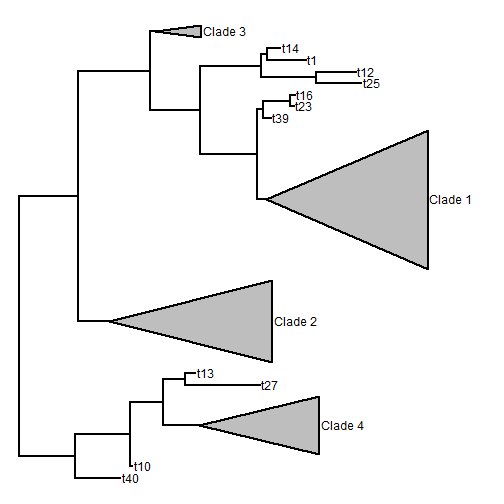``````cols ## color some of the clades
``````
``````##  Clade 1  Clade 2  Clade 4
##  "black"    "red" "green3"
``````
``````par(cex=0.8) ## change font size
par(font=3) ## change font type
plot(obj,col=cols,vscale=0.7)
``````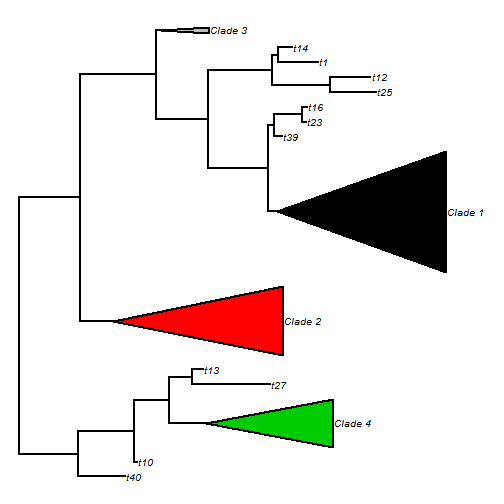Here is the interactive demo:

## Saturday, June 11, 2016

### Update to `roundPhylogram` to permit different `lwd` and `lty` for different edges

I just pushed a small update to `roundPhylogram` that permits different line types (`lty`) and line widths (`lwd`) to be specified for different branches of the tree. This will be done whenever either argument is supplied as a vector instead of as a single scalar value or (in the case of `lty`) as a character string.

I did this so that I could plot taxa with ambiguous phylogenetic placement (say) using a dotted line. Note that it would also be straightforward to do this in functions using `plotSimmap` or the internal function `phylogram`, but this does not yet exist. It may exist in the generic S3 `plot` method for objects of class `"phylo"`. I don't know, but I wouldn't be surprised.

Here is what I mean.

``````library(phytools)
packageVersion("phytools") ## latest GitHub version
``````
``````##  '0.5.37'
``````
``````## read trees
t1<-"((((A:0.5,D:0.5):0.5,B:1):1,C:2):1,E:3);"
t2<-"(((A:1,B:1):1,C:2):1,(D:0.5,E:0.5):2.5);"

par(mfrow=c(2,1))

lty<-rep("solid",nrow(t1\$edge))
lty[which(t1\$edge[,2]==which(t1\$tip.label=="D"))]<-
"dashed"
roundPhylogram(t1,mar=c(0.1,0.1,3.1,0.1),
lty=lty,lwd=3)
lty<-rep("solid",nrow(t2\$edge))
lty[which(t2\$edge[,2]==which(t2\$tip.label=="D"))]<-
"dashed"
roundPhylogram(t2,mar=c(0.1,0.1,3.1,0.1),
lty=lty,lwd=3)
``````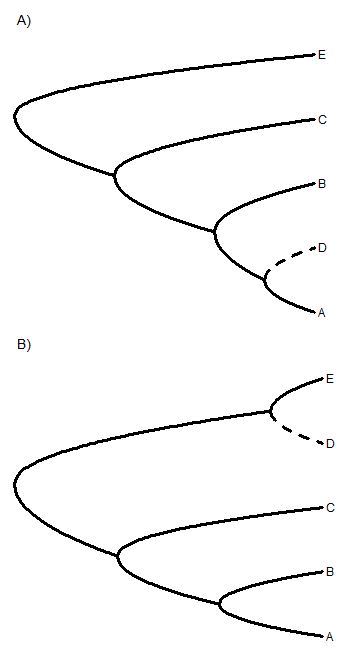Note that the consensus of these two trees (similar as they are in so many respects, except with respect to the placement of `"D"`), is a star tree:

``````cons<-consensus(c(t1,t2))
lty<-rep("solid",nrow(cons\$edge))
lty[which(cons\$edge[,2]==which(cons\$tip.label=="D"))]<-
"dashed"
roundPhylogram(cons,lty=lty)
``````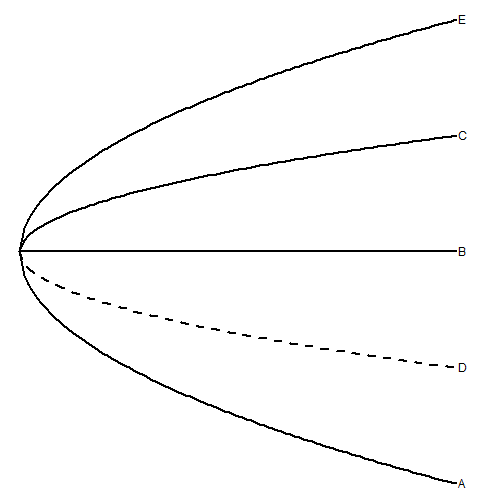## Tuesday, June 7, 2016

### Fix to `contMap` for short edges descended from the root node

A phytools user, Bruno Vilela, recently reported the bug that the phytools function `contMap` can fail for very short internal edges. This tends to be the case when an edge has a length that is less than `1/res` × the tree height, in which `res` is an argument of `contMap` specifying the graininess of the color gradient to be plotted along edges.

[Actually, I believe I already fixed this bug except for edges descended from the root node. This is because for those edges the function will attempt to create a list of mappings along edges, but create a vector instead. Silly!]

I have previously suggested collapsing very short internal edges or increasing `res`; however Bruno did one better by providing an incredibly simple solution, which was to create a list of mappings along edges before iterating across the edges of the tree to compute the mappings. Perhaps obvious in retrospect, please keep in mind that I wrote the first version of this function in 2012 when I was quite an R programming novice. (I'm not sure what I'd call myself now. Slightly more than an a novice? I guess in an era when Donald Trump is running for present I'm a world-class R programming expert!)

Here's a quick demo of the problem, along with a demonstration that Bruno's fix does indeed restore function:

``````library(phytools)
tree
``````
``````##
## Phylogenetic tree with 26 tips and 25 internal nodes.
##
## Tip labels:
##  t5, t26, t18, t17, t19, t22, ...
##
## Rooted; includes branch lengths.
``````
``````x
``````
``````##          t5         t26         t18         t17         t19         t22
##  0.20063042  0.90298749  2.28555569  2.11331867  1.38012423  0.01110355
##         t15         t12          t6          t3          t2         t24
##  0.31029532 -0.31270146  0.36006396  0.86328160 -0.16281977 -1.88665386
##          t4          t9         t25         t11          t8         t21
## -2.40507772 -2.69645998 -1.57485299  1.30619095  1.96062919 -0.91760839
##          t1         t14          t7         t16         t20         t23
## -1.45220994 -2.58959187 -1.44919866  0.93419977  0.19530914  0.06853757
##         t13         t10
##  1.79467390  0.29857041
``````
``````plotTree(tree) ## short node from the root
``````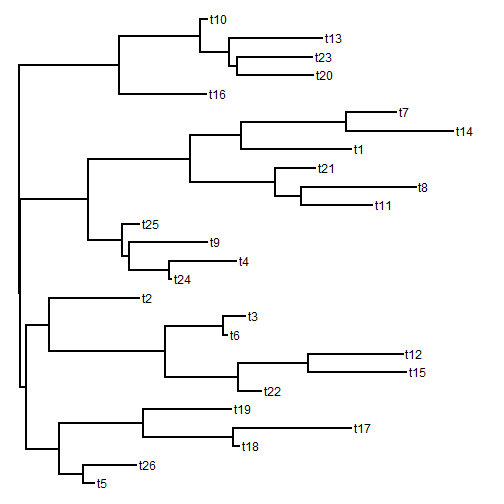``````obj<-contMap(tree,x,plot=FALSE)
``````
``````## Error in tree\$maps[[i]] <- XX[, 2] - XX[, 1]: more elements supplied than there are to replace
``````
``````## increase res
obj<-contMap(tree,x,plot=FALSE,res=1000)
plot(obj)
``````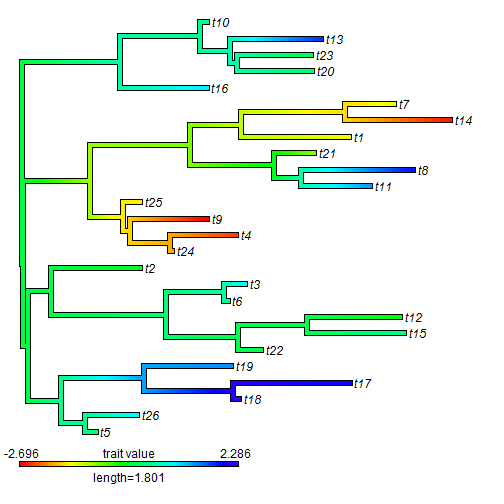Now let's load the new version from source off GitHub:

``````source("https://raw.githubusercontent.com/liamrevell/phytools/master/R/contMap.R")
source("https://raw.githubusercontent.com/liamrevell/phytools/master/R/densityMap.R")
obj<-contMap(tree,x,plot=FALSE)
plot(obj)
``````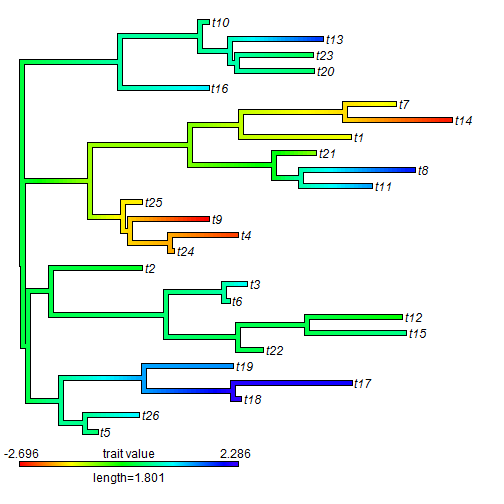This fix also allows us to set the value of `res` to any arbitrarily low value. Although the resultant plot is grainier, this might nonetheless be useful for users working with very large trees where computation is an issue.

``````contMap(tree,x,res=100)
````````````contMap(tree,x,res=10)
``````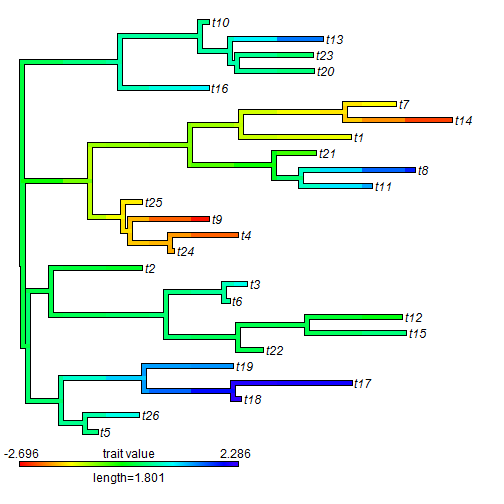Thanks Bruno!